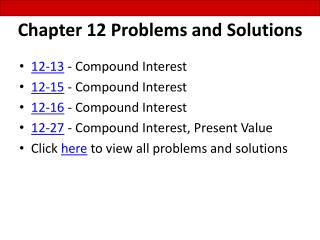DownloadDownload PresentationChapter 12 Problems and Solutions

# Chapter 12 Problems and Solutions

Télécharger la présentation## Chapter 12 Problems and Solutions

- - - - - - - - - - - - - - - - - - - - - - - - - - - E N D - - - - - - - - - - - - - - - - - - - - - - - - - - -
##### Presentation Transcript

1. 12-13 - Compound Interest 12-15 - Compound Interest 12-16 - Compound Interest 12-27 - Compound Interest, Present Value Click here to view all problems and solutions Chapter 12 Problems and Solutions

2. Problem 12-13 Lynn Ally, owner of a local Subway shop, loaned \$40,000 to Pete Hall to help him open a Subway franchise. Pete plans to repay Lynn at the end of 8 years with 6% interest compounded semiannually. How much will Lynn receive at the end of 8 years? Step 1: Calculate the number of periods. 8 years x 2 periods per year = 16 periods Step 2: Calculate the interest rate per period. 6% = 3% 2 Step 3: Look up the table factor on the future value of \$1 table. 1.6047 is at the intersection of 16 periods @ 3% Step 4: Calculate the future value Lynn will receive. \$40,000 x 1.6047 = \$64,188

3. Problem 12-15 Melvin Indecision has difficulty deciding whether to put his savings in Mystic Bank or Four Rivers Bank. Mystic offers 10% interest compounded semiannually. Four Rivers offers 8% interest compounded quarterly. Melvin has \$10,000 to invest. He expects to withdraw the money at the end of 4 years. Which bank gives Melvin the better deal? Step 1: Calculate the number of periods for both banks. Mystic 4 years x 2 periods per year = 8 periods Four Rivers 4 years x 4 periods per year = 16 periods Step 2: Calculate the interest rate per period for both banks. Mystic 10% = 5% 2 Four Rivers 8% = 2% 4 Step 3: Look up the table factor on the future value of \$1 table. Mystic 1.4775 is at the intersection of 8 periods @ 5% Four Rivers 1.3728 is at the intersection of 16 periods @ 2%

4. Problem 12-15 continued Step 4: Calculate the future value at both banks. Mystic \$10,000 x 1.4775 = \$14,775 Four Rivers \$10,000 x 1.3728 = \$13,728 Step 5: Calculate interest earned at both banks. Mystic \$14,775 – 10,000 = \$4,775 Four Rivers \$13,728 – 10,000 = \$3,728 Step 6: Choose the bank with the greatest interest earned. Mystic Bank has the better deal by \$1,047 (\$4,775 - \$3,728).

5. Problem 12-16 Lee Holmes deposited \$15,000 in a new savings account at 9% interest compounded semiannually. At the beginning of year 4, Lee deposits an additional \$40,000 at 9% compounded semiannually. At the end of 6 years, what is the balance in Lee’s account? Step 1: Calculate the number of periods. 3 years x 2 periods per year = 6 periods Step 2: Calculate the interest rate per period. 9% = 4 ½ % 2 Step 3: Look up the table factor on the future value of \$1 table. 1.3023 is at the intersection of 6 periods @ 4 ½ % Step 4: Calculate the future value. \$15,000 x 1.3023 = \$19,534.50

6. Problem 12-16 continued Step 5: Add the \$40,000 deposit to the future value amount. \$19,534.50 + 40,000 = \$59,534.50 Step 6: Calculate the number of periods. 3 years x 2 periods per year = 6 periods Step 7: Calculate the interest rate per period. 9% = 4 ½ % 2 Step 8: Look up the table factor on the future value of \$1 table. 1.3023 is at the intersection of 6 periods @ 4 ½ % Step 9: Calculate the future value. \$59,534.50 x 1.3023 = \$77,531.78

7. Problem 12-27 Paul Havlik promised his grandson Jamie that he would give him \$6,000 8 years from today for graduating from high school. Assume money is worth 6% interest compounded semiannually. What is the present value of this \$6,000? Step 1: Calculate the number of periods. 8 years x 2 periods per year = 16 periods Step 2: Calculate the interest rate per period. 6% = 3% 2 Step 3: Look up the table factor on the present value of \$1 table. .6232 is at the intersection of 16 periods @ 3% Step 4: Calculate the present value of this investment. \$6,000 x .6232 = \$3,739.20